Share

NCERT solutions for Class 12 Physics chapter 1 - Electric Charge and Fields

Physics Textbook for Class 12

NCERT Physics Class 12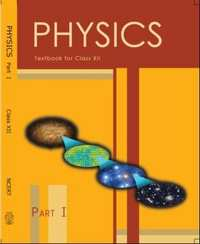Chapter 1: Electric Charge and Fields

Chapter 1: Electric Charge and Fields solutions [Pages 46 - 50]

Q 1 | Page 46

What is the force between two small charged spheres having charges of 2 × 10−7 C and 3 × 10−7 C placed 30 cm apart in air?

Q 2 | Page 46

The electrostatic force on a small sphere of charge 0.4 μC due to another small sphere of charge − 0.8 μC in air is 0.2 N. (a) What is the distance between the two spheres? (b) What is the force on the second sphere due to the first?

Q 3 | Page 46

Check that the ratio ke2/G memp is dimensionless. Look up a Table of Physical Constants and determine the value of this ratio. What does the ratio signify?

Q 4.1 | Page 46

Explain the meaning of the statement ‘electric charge of a body is quantised’

Q 4.1 | Page 46

Why can one ignore quantisation of electric charge when dealing with macroscopic i.e., large scale charges?

Q 5 | Page 46

When a glass rod is rubbed with a silk cloth, charges appear on both. A similar phenomenon is observed with many other pairs of bodies. Explain how this observation is consistent with the law of conservation of charge.

Q 6 | Page 46

Four point charges q= 2 μC, q= −5 μC, qC = 2 μC, and qD = −5 μC are located at the corners of a square ABCD of side 10 cm. What is the force on a charge of 1 μC placed at the centre of the square?

Q 7 | Page 46

An electrostatic field line is a continuous curve. That is, a field line cannot have sudden breaks. Why not?

Q 7.2 | Page 46

Why do the electric field lines never cross each other?

Q 8 | Page 46

Two point charges qA = 3 μC and qB = −3 μC are located 20 cm apart in vacuum.

(a) What is the electric field at the midpoint O of the line AB joining the two charges?

(b) If a negative test charge of magnitude 1.5 × 10−9 C is placed at this point, what is the force experienced by the test charge?

Q 9 | Page 46

A system has two charges qA = 2.5 × 10−7 C and qB = −2.5 × 10−7 C located at points A: (0, 0, − 15 cm) and B: (0, 0, + 15 cm), respectively. What are the total charge and electric dipole moment of the system?

Q 10 | Page 46

An electric dipole with dipole moment 4 × 10−9 C m is aligned at 30° with the direction of a uniform electric field of magnitude 5 × 104 N C−1. Calculate the magnitude of the torque acting on the dipole.

Q 11 | Page 46

A polythene piece rubbed with wool is found to have a negative charge of 3 × 10−7 C.

(a) Estimate the number of electrons transferred (from which to which?)

(b) Is there a transfer of mass from wool to polythene?

Q 12.1 | Page 46

Two insulated charged copper spheres A and B have their centers separated by a distance of 50 cm. What is the mutual force of electrostatic repulsion if the charge on each is 6.5 × 10−7 C? The radii of A and B are negligible compared to the distance of separation

Q 12.2 | Page 47

What is the force of repulsion if each sphere is charged double the above amount, and the distance between them is halved?

Q 13 | Page 47

Suppose the spheres A and B in Exercise 1.12 have identical sizes. A third sphere of the same size but uncharged is brought in contact with the first, then brought in contact with the second, and finally removed from both. What is the new force of repulsion between A and B?

Q 14 | Page 47

The figure shows tracks of three charged particles in a uniform electrostatic field. Give the signs of the three charges. Which particle has the highest charge to mass ratio?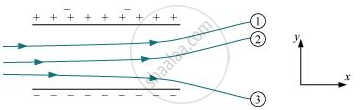Q 15 | Page 47

Consider a uniform electric field E = 3 × 103 îN/C. (a) What is the flux of this field through a square of 10 cm on a side whose plane is parallel to the yz plane? (b) What is the flux through the same square if the normal to its plane makes a 60° angle with the x-axis?

Q 16 | Page 47

What is the net flux of the uniform electric field of Exercise 1.15 through a cube of side 20 cm oriented so that its faces are parallel to the coordinate planes?

Q 17 | Page 47

Careful measurement of the electric field at the surface of a black box indicates that the net outward flux through the surface of the box is 8.0 × 103 N m2/C. (a) What is the net charge inside the box? (b) If the net outward flux through the surface of the box were zero, could you conclude that there were no charges inside the box? Why or Why not?

Q 18 | Page 47

A point charge +10 μC is a distance 5 cm directly above the centre of a square of side 10 cm, as shown in Fig. 1.34. What is the magnitude of the electric flux through the square? (Hint: Think of the square as one face of a cube with edge 10 cm.)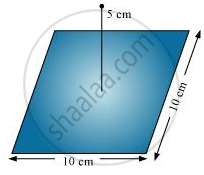Q 19 | Page 48

A point charge of 2.0 μC is at the centre of a cubic Gaussian surface 9.0 cm on edge. What is the net electric flux through the surface?

Q 20 | Page 48

A point charge causes an electric flux of −1.0 × 103 Nm2/C to pass through a spherical Gaussian surface of 10.0 cm radius centred on the charge. (a) If the radius of the Gaussian surface were doubled, how much flux would pass through the surface? (b) What is the value of the point charge?

Q 21 | Page 48

A conducting sphere of radius 10 cm has an unknown charge. If the electric field 20 cm from the centre of the sphere is 1.5 × 10N/C and points radially inward, what is the net charge on the sphere?

Q 22 | Page 48

A uniformly charged conducting sphere of 2.4 m diameter has a surface charge density of 80.0 μC/m2. (a) Find the charge on the sphere. (b) What is the total electric flux leaving the surface of the sphere?

Q 23 | Page 48

An infinite line charge produces a field of 9 × 104 N/C at a distance of 2 cm. Calculate the linear charge density.

Q 24 | Page 48

Two large, thin metal plates are parallel and close to each other. On their inner faces, the plates have surface charge densities of opposite signs and of magnitude 17.0 × 10−22 C/m2. What is E: (a) in the outer region of the first plate, (b) in the outer region of the second plate, and (c) between the plates?

Q 25 | Page 48

An oil drop of 12 excess electrons is held stationary under a constant electric field of 2.55 × 104 N C−1in Millikan’s oil drop experiment. The density of the oil is 1.26 g cm−3. Estimate the radius of the drop. (= 9.81 m s−2= 1.60 × 10−19 C).

Q 26 | Page 48

Which among the curves shown in Fig. 1.35 cannot possibly represent electrostatic field lines?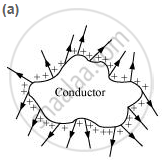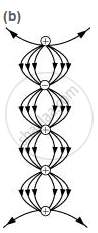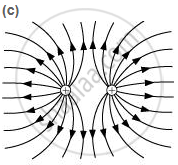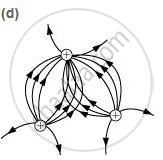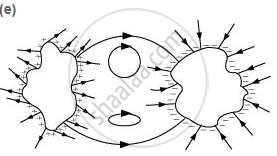Q 27 | Page 49

In a certain region of space, electric field is along the z-direction throughout. The magnitude of electric field is, however, not constant but increases uniformly along the positive z-direction, at the rate of 105NC−1 per metre. What are the force and torque experienced by a system having a total dipole moment equal to 10−7 Cm in the negative z-direction?

Q 28 | Page 49

(a) A conductor A with a cavity as shown in Fig. 1.36(a) is given a charge Q. Show that the entire charge must appear on the outer surface of the conductor. (b) Another conductor B with charge is inserted into the cavity keeping B insulated from A. Show that the total charge on the outside surface of A is [Fig. 1.36(b)]. (c) A sensitive instrument is to be shielded from the strong electrostatic fields in its environment. Suggest a possible way.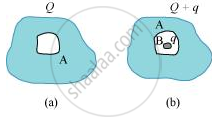Q 29 | Page 49

A hollow charged conductor has a tiny hole cut into its surface. Show that the electric field in the hole is(sigma/(2in_0)) hatn. where hatn is the unit vector in the outward normal direction, and sigma is the surface charge density near the hole.

Q 30 | Page 49

Obtain the formula for the electric field due to a long thin wire of uniform linear charge density λ without using Gauss’s law. [Hint: Use Coulomb’s law directly and evaluate the necessary integral.]

Q 31 | Page 49

It is now believed that protons and neutrons (which constitute nuclei of ordinary matter) are themselves built out of more elementary units called quarks. A proton and a neutron consist of three quarks each. Two types of quarks, the so called ‘up’ quark (denoted by u) of charge (+2/3) e, and the ‘down’ quark (denoted by d) of charge (−1/3) e, together with electrons build up ordinary matter. (Quarks of other types have also been found which give rise to different unusual varieties of matter.) Suggest a possible quark composition of a proton and neutron.

Q 32 | Page 50

(a) Consider an arbitrary electrostatic field configuration. A small test charge is placed at a null point (i.e., where = 0) of the configuration. Show that the equilibrium of the test charge is necessarily unstable.

(b) Verify this result for the simple configuration of two charges of the same magnitude and sign placed a certain distance apart.

Q 33 | Page 50

A particle of mass and charge (−q) enters the region between the two charged plates initially moving along x-axis with speed vx (like particle 1 in Fig. 1.33). The length of plate is and an uniform electric field is maintained between the plates. Show that the vertical deflection of the particle at the far edge of the plate is qEL2/ (2mv_s^2).

Q 34 | Page 50

Suppose that the particle in Exercise in 1.33 is an electron projected with velocity vx= 2.0 × 106 m s−1. If between the plates separated by 0.5 cm is 9.1 × 102 N/C, where will the electron strike the upper plate? (| | =1.6 × 10−19 C, m= 9.1 × 10−31 kg.)

Chapter 1: Electric Charge and Fields Extra questions

If Coulomb’s law involved 1/r3 dependence (instead of 1/r2), would Gauss’s law be still true?

Chapter 1: Electric Charge and Fields

NCERT Physics Class 12NCERT solutions for Class 12 Physics chapter 1 - Electric Charge and Fields

NCERT solutions for Class 12 Physics chapter 1 (Electric Charge and Fields) include all questions with solution and detail explanation. This will clear students doubts about any question and improve application skills while preparing for board exams. The detailed, step-by-step solutions will help you understand the concepts better and clear your confusions, if any. Shaalaa.com has the CBSE Physics Textbook for Class 12 solutions in a manner that help students grasp basic concepts better and faster.

Further, we at Shaalaa.com are providing such solutions so that students can prepare for written exams. NCERT textbook solutions can be a core help for self-study and acts as a perfect self-help guidance for students.

Concepts covered in Class 12 Physics chapter 1 Electric Charge and Fields are Gauss’s Law, Physical Significance of Electric Field, Electric Field Due to a System of Charges, Charging by Induction, Electric Field Due to a Point Charge, Uniformly Charged Infinite Plane Sheet and Uniformly Charged Thin Spherical Shell (Field Inside and Outside), Applications of Gauss’s Law, Electric Flux, Dipole in a Uniform External Field, Electric Dipole, Electric Field Lines, Introduction of Electric Field, Continuous Charge Distribution, Superposition Principle of Forces, Forces Between Multiple Charges, Force Between Two Point Charges, Coulomb’s Law, Basic Properties of Electric Charge, Electric Charges.

Using NCERT Class 12 solutions Electric Charge and Fields exercise by students are an easy way to prepare for the exams, as they involve solutions arranged chapter-wise also page wise. The questions involved in NCERT Solutions are important questions that can be asked in the final exam. Maximum students of CBSE Class 12 prefer NCERT Textbook Solutions to score more in exam.

Get the free view of chapter 1 Electric Charge and Fields Class 12 extra questions for Physics and can use Shaalaa.com to keep it handy for your exam preparation

S